# Introduction

Here I show how to produce P-value, S-value, likelihood, and deviance functions with the concurve package using fake data and data from real studies. Simply put, these functions are rich sources of information for scientific inference and the image below, taken from Xie & Singh, 20131 displays why.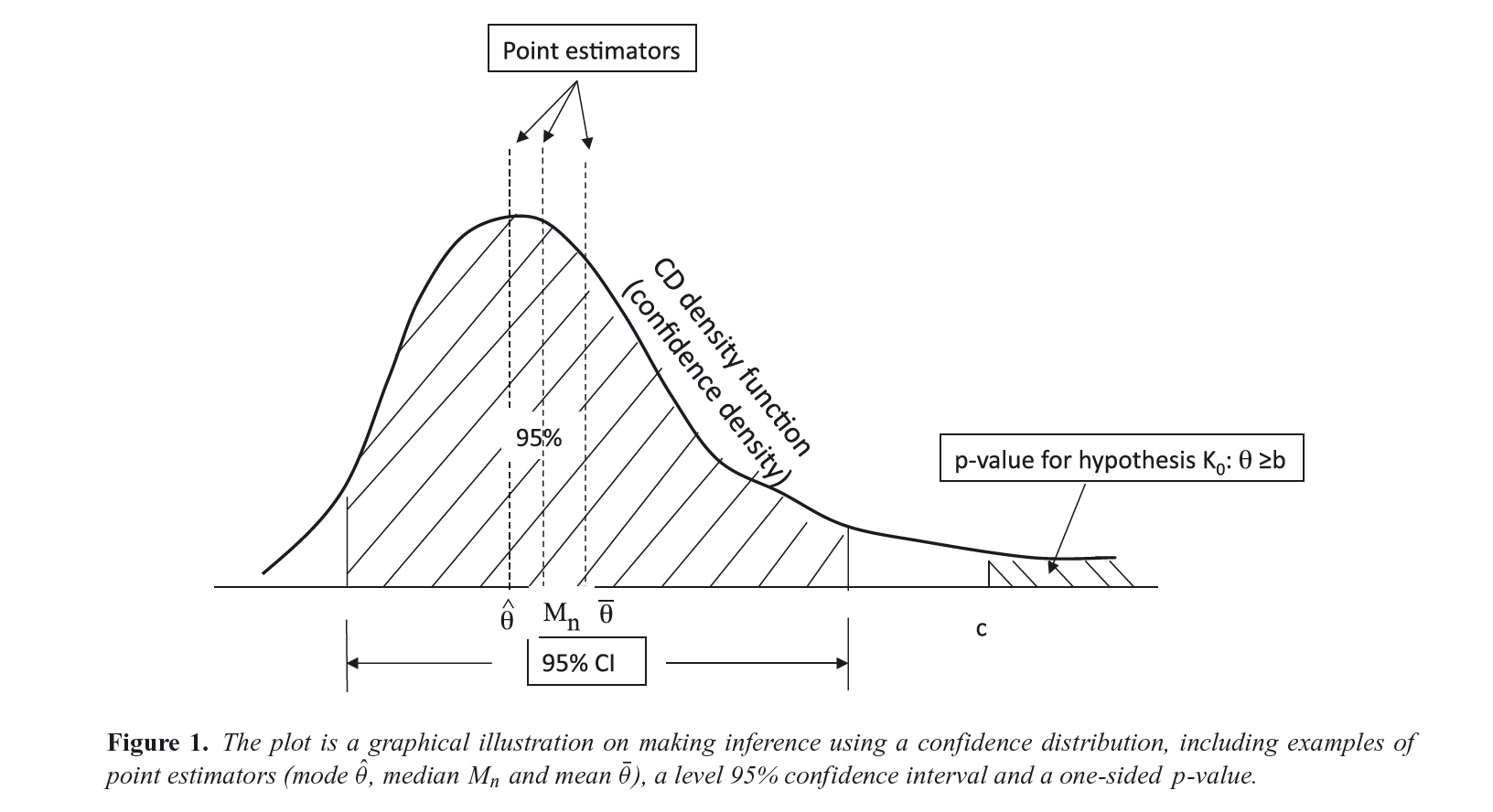For a more extensive discussion of these concepts, see the following references.113

# Simple Models

To get started, we could generate some normal data and combine two vectors in a dataframe

library(concurve)
set.seed(1031)
GroupA <- rnorm(500)
GroupB <- rnorm(500)
RandomData <- data.frame(GroupA, GroupB)

and look at the differences between the two vectors. We’ll plug these vectors and the dataframe they’re in inside of the curve_mean() function. Here, the default method involves calculating CIs using the Wald method.

intervalsdf <- curve_mean(GroupA, GroupB,
data = RandomData, method = "default"
)

Each of the functions within concurve will generally produce a list with three items, and the first will usually contain the function of interest.

head(intervalsdf[], 10)
#>    lower.limit upper.limit intrvl.width intrvl.level     cdf pvalue
#> 1   -0.1125581  -0.1125581 0.000000e+00        0e+00 0.50000 1.0000
#> 2   -0.1125658  -0.1125504 1.543412e-05        1e-04 0.50005 0.9999
#> 3   -0.1125736  -0.1125427 3.086824e-05        2e-04 0.50010 0.9998
#> 4   -0.1125813  -0.1125350 4.630236e-05        3e-04 0.50015 0.9997
#> 5   -0.1125890  -0.1125273 6.173649e-05        4e-04 0.50020 0.9996
#> 6   -0.1125967  -0.1125195 7.717061e-05        5e-04 0.50025 0.9995
#> 7   -0.1126044  -0.1125118 9.260473e-05        6e-04 0.50030 0.9994
#> 8   -0.1126122  -0.1125041 1.080389e-04        7e-04 0.50035 0.9993
#> 9   -0.1126199  -0.1124964 1.234730e-04        8e-04 0.50040 0.9992
#> 10  -0.1126276  -0.1124887 1.389071e-04        9e-04 0.50045 0.9991
#>          svalue
#> 1  0.0000000000
#> 2  0.0001442767
#> 3  0.0002885679
#> 4  0.0004328734
#> 5  0.0005771935
#> 6  0.0007215279
#> 7  0.0008658768
#> 8  0.0010102402
#> 9  0.0011546179
#> 10 0.0012990102

We can view the function using the ggcurve() function. The two basic arguments that must be provided are the data argument and the “type” argument. To plot a consonance function, we would write “c”.

(function1 <- ggcurve(data = intervalsdf[], type = "c", nullvalue = TRUE))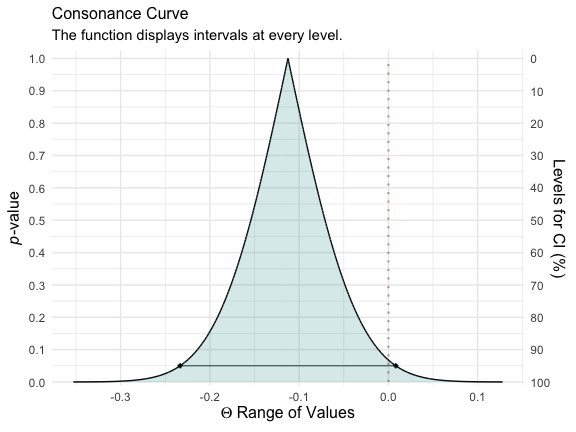We can see that the consonance “curve” is every interval estimate plotted, and provides the P-values, CIs, along with the median unbiased estimate It can be defined as such,

$C V_{n}(\theta)=1-2\left|H_{n}(\theta)-0.5\right|=2 \min \left\{H_{n}(\theta), 1-H_{n}(\theta)\right\}$

Its information counterpart, the surprisal function, can be constructed by taking the $$-log_{2}$$ of the P-value.3,14,15

To view the surprisal function, we simply change the type to “s”.

(function1 <- ggcurve(data = intervalsdf[], type = "s"))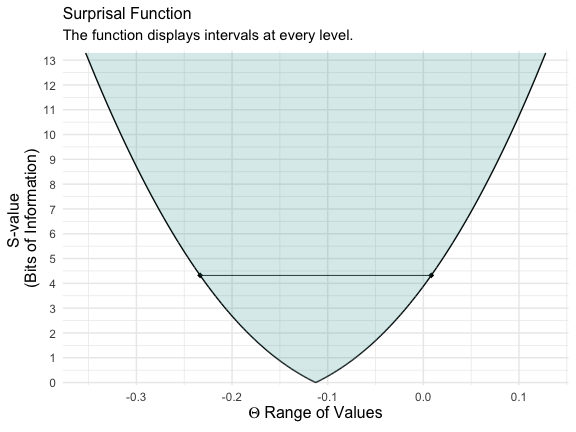We can also view the consonance distribution by changing the type to “cdf”, which is a cumulative probability distribution. The point at which the curve reaches 50% is known as the “median unbiased estimate”. It is the same estimate that is typically at the peak of the P-value curve from above.

(function1s <- ggcurve(data = intervalsdf[], type = "cdf", nullvalue = TRUE))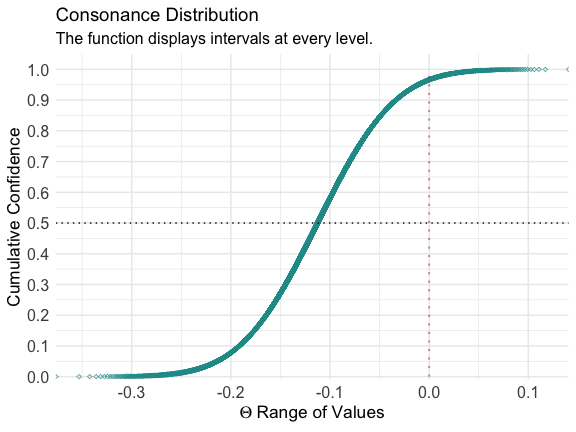We can also get relevant statistics that show the range of values by using the curve_table() function. There are several formats that can be exported such as .docx, .ppt, and TeX.

(x <- curve_table(data = intervalsdf[], format = "image"))
 Lower Limit Upper Limit Interval Width Interval Level (%) CDF P-value S-value (bits) -0.132 -0.093 0.039 25.0 0.625 0.750 0.415 -0.154 -0.071 0.083 50.0 0.750 0.500 1.000 -0.183 -0.042 0.142 75.0 0.875 0.250 2.000 -0.192 -0.034 0.158 80.0 0.900 0.200 2.322 -0.201 -0.024 0.177 85.0 0.925 0.150 2.737 -0.214 -0.011 0.203 90.0 0.950 0.100 3.322 -0.233 0.008 0.242 95.0 0.975 0.050 4.322 -0.251 0.026 0.276 97.5 0.988 0.025 5.322 -0.271 0.046 0.318 99.0 0.995 0.010 6.644

# Comparing Functions

If we wanted to compare two studies to see the amount of “consonance”, we could use the curve_compare() function to get a numerical output.

First, we generate some more fake data

GroupA2 <- rnorm(500)
GroupB2 <- rnorm(500)
RandomData2 <- data.frame(GroupA2, GroupB2)
model <- lm(GroupA2 ~ GroupB2, data = RandomData2)
randomframe <- curve_gen(model, "GroupB2")

Once again, we’ll plot this data with ggcurve(). We can also indicate whether we want certain interval estimates to be plotted in the function with the “levels” argument. If we wanted to plot the 50%, 75%, and 95% intervals, we’d provide the argument this way:

(function2 <- ggcurve(type = "c", randomframe[], levels = c(0.50, 0.75, 0.95), nullvalue = TRUE))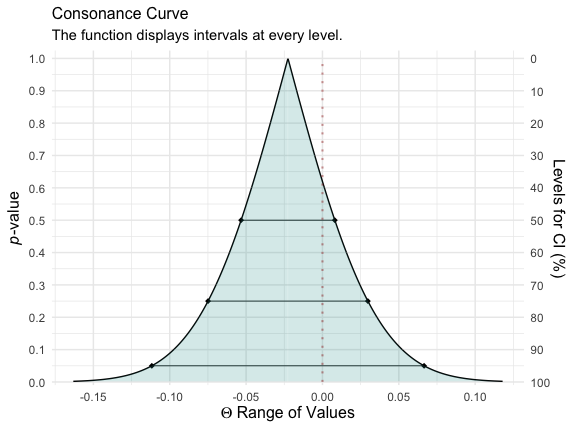Now that we have two datasets and two functions, we can compare them using the curve_compare() function.

(curve_compare(
data1 = intervalsdf[], data2 = randomframe[], type = "c",
plot = TRUE, measure = "default", nullvalue = TRUE
))
#>  "AUC = Area Under the Curve"
#> []
#>
#>
#>  AUC 1   AUC 2   Shared AUC   AUC Overlap (%)   Overlap:Non-Overlap AUC Ratio
#> ------  ------  -----------  ----------------  ------------------------------
#>  0.098   0.073        0.024            16.309                           0.195
#>
#> []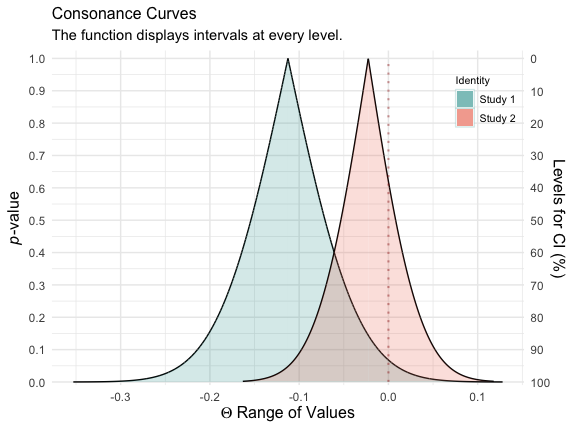This function will provide us with the area that is shared between the curve, along with a ratio of overlap to non-overlap.

Another way to compare the functions is to use the cowplot plot_grid() function.

cowplot::plot_grid(function1, function2)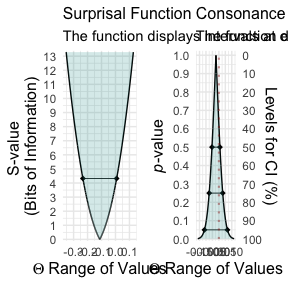We can also do this for the surprisal function simply by changing type to “s”.

(curve_compare(
data1 = intervalsdf[], data2 = randomframe[], type = "s",
plot = TRUE, measure = "default", nullvalue = FALSE
))
#>  "AUC = Area Under the Curve"
#> []
#>
#>
#>  AUC 1   AUC 2   Shared AUC   AUC Overlap (%)   Overlap:Non-Overlap AUC Ratio
#> ------  ------  -----------  ----------------  ------------------------------
#>  3.947   1.531        1.531            38.801                           0.634
#>
#> []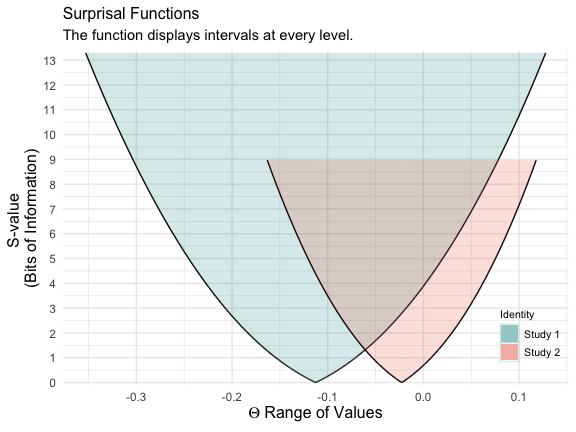It’s clear that the outputs have changed and indicate far more overlap than before.

# Constructing Functions From Single Intervals

We can also take a set of confidence limits and use them to construct a consonance, surprisal, likelihood or deviance function using the curve_rev() function. This method is computed from the approximate normal distribution.

Here, we’ll use two epidemiological studies16,17 that studied the impact of SSRI exposure in pregnant mothers, and the rate of autism in children.

Both of these studies suggested a null effect of SSRI exposure on autism rates in children.

curve1 <- curve_rev(point = 1.7, LL = 1.1, UL = 2.6, type = "c", measure = "ratio", steps = 10000)
(ggcurve(data = curve1[], type = "c", measure = "ratio", nullvalue = TRUE))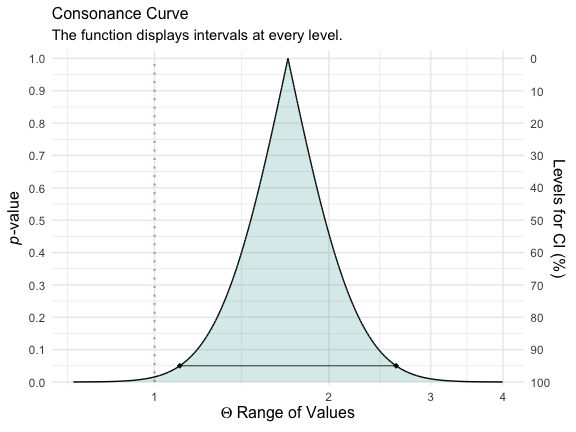curve2 <- curve_rev(point = 1.61, LL = 0.997, UL = 2.59, type = "c", measure = "ratio", steps = 10000)
(ggcurve(data = curve2[], type = "c", measure = "ratio", nullvalue = TRUE))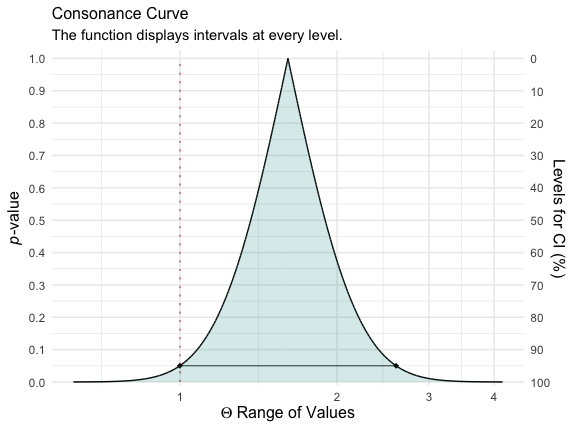The null value is shown via the red line and it’s clear that a large mass of the function is away from it.

We can also see this by plotting the likelihood functions via the curve_rev() function.

lik1 <- curve_rev(point = 1.7, LL = 1.1, UL = 2.6, type = "l", measure = "ratio", steps = 10000)
(ggcurve(data = lik1[], type = "l1", measure = "ratio", nullvalue = TRUE))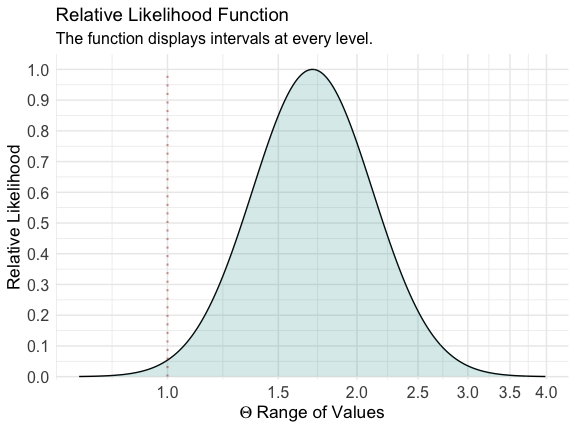lik2 <- curve_rev(point = 1.61, LL = 0.997, UL = 2.59, type = "l", measure = "ratio", steps = 10000)
(ggcurve(data = lik2[], type = "l1", measure = "ratio", nullvalue = TRUE))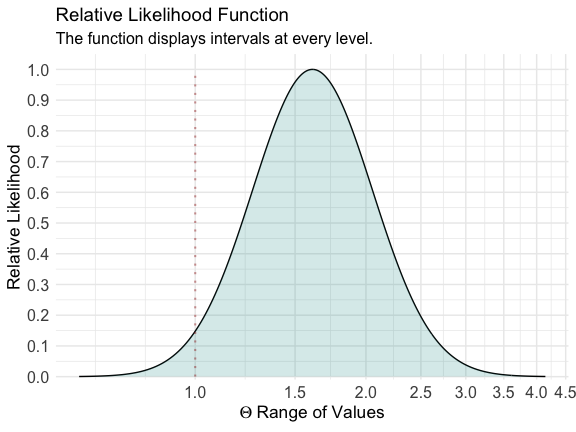We can also view the amount of agreement between the likelihood functions of these two studies.

(plot_compare(
data1 = lik1[], data2 = lik2[], type = "l1", measure = "ratio", nullvalue = TRUE, title = "Brown et al. 2017. J Clin Psychiatry. vs. \nBrown et al. 2017. JAMA.",
subtitle = "J Clin Psychiatry: OR = 1.7, 1/6.83 LI: LL = 1.1, UL = 2.6 \nJAMA: HR = 1.61, 1/6.83 LI: LL = 0.997, UL = 2.59", xaxis = expression(Theta ~ "= Hazard Ratio / Odds Ratio")
))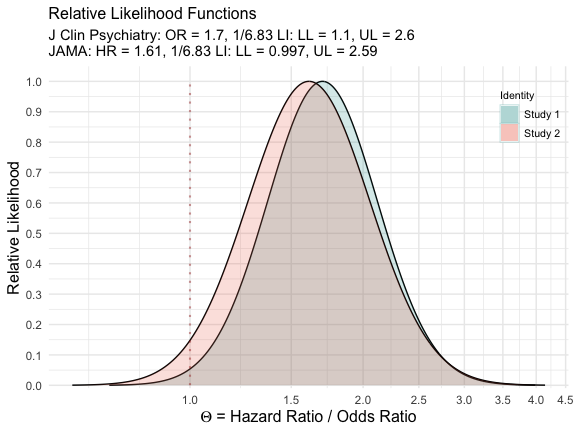and the consonance functions

(plot_compare(
data1 = curve1[], data2 = curve2[], type = "c", measure = "ratio", nullvalue = TRUE, title = "Brown et al. 2017. J Clin Psychiatry. vs. \nBrown et al. 2017. JAMA.",
subtitle = "J Clin Psychiatry: OR = 1.7, 1/6.83 LI: LL = 1.1, UL = 2.6 \nJAMA: HR = 1.61, 1/6.83 LI: LL = 0.997, UL = 2.59", xaxis = expression(Theta ~ "= Hazard Ratio / Odds Ratio")
))